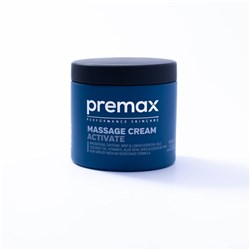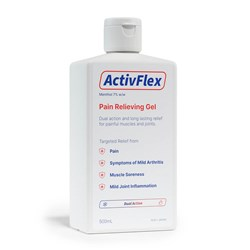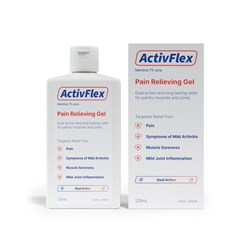# Topical Products

Grid List
\$49.90
Increase value Decrease value
\$16.60
Increase value Decrease value\$39.00
Increase value Decrease value
\$29.99
Increase value Decrease value
\$29.99
Increase value Decrease value
\$29.99
Increase value Decrease value
\$24.95
Increase value Decrease value
\$29.99
Increase value Decrease value
\$29.99
Increase value Decrease value
\$29.00
Increase value Decrease value
\$20.50
Increase value Decrease value
\$39.00
Increase value Decrease value
\$39.00
Increase value Decrease value
\$39.00
Increase value Decrease value
\$38.50
Increase value Decrease value
\$79.90
Increase value Decrease value
QV Cream 1kg
\$66.00
Increase value Decrease value
QV Heel Balm (50g)
\$16.87
Increase value Decrease value
Bio Oil 200ml
\$57.42
Increase value Decrease value\$37.99
Increase value Decrease value\$24.99
Increase value Decrease value
SolvEasy Tinea Cream 15g
\$18.48
Increase value Decrease value
Baby Oil 200ml
In Stock
\$12.75
Increase value Decrease value
\$8.10
Increase value Decrease value
\$33.02
Increase value Decrease value
\$47.51
Increase value Decrease value
\$56.95
Increase value Decrease value
\$228.50
Increase value Decrease value
\$8.54
Increase value Decrease value
\$18.60
Increase value Decrease value
\$24.95
Increase value Decrease value
From
Increase value Decrease value
\$21.70
\$157.20
\$16.17
Increase value Decrease value
Tiger Balm White 18g
\$16.63
Increase value Decrease value
\$35.90
\$58.01
Increase value Decrease value
\$26.20
Increase value Decrease value
\$13.23
Increase value Decrease value
\$24.85
Increase value Decrease value
\$48.69
Increase value Decrease value
\$28.25
Increase value Decrease value
\$9.20
Increase value Decrease value
\$21.10
Increase value Decrease value
\$11.00
Increase value Decrease value
\$16.34
Increase value Decrease value
\$19.21
Increase value Decrease value
Grid List
##### Products to compare:
Comparing Products